# The SORT Function for Data Analytics in Excel## The SORT Function for Data Analytics in Excel

In our last blog, we discussed the new Unique function in Excel. In this blog, we will discuss about the newly introduced Sort Function, which is also useful for analyzing large data sets. This function has been introduced in Microsoft Excel 365. It is not available in earlier versions of excel.

The Sort function helps sort a range or an array dynamically. The Sort feature available earlier helped sort data or ranges but was not a dynamic feature. In case of any change in data, the sorting had to be done again using the Sort feature. This is not the case with the new Sort function.

## Sort Function Arguments

The Sort Function has 4 arguments.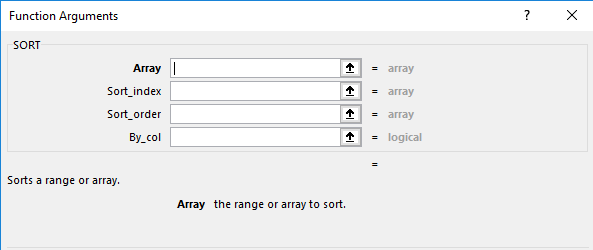Array :- It is the range or array to be sorted

Sort_Index :- It is the row or column in the dataset to be sorted. The input for this argument is a number.

Sort_Order :- It is the desired sort order. The input is a number. 1 represents Ascending order and -1 represents Descending Order.

By_Col :- In most cases, the values are sorted by the rows. The default value for this argument is “False”. So, if we do not enter anything for this argument, excel would sort the values by the rows in a selected column. If we want to sort the values by column (rare situations), we should enter “True” in the argument.

Let us understand the Sort Function with the help of a few examples.

Click to learn top 10 financial functions in Excel

### Example 1 – Sorting a List of Names ascending order

Here is a list of customer names who visited the store this week and sales made.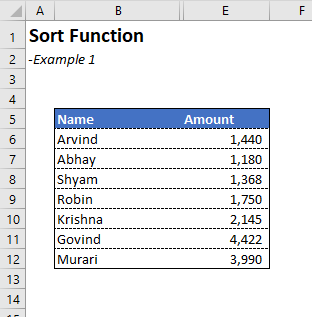To sort the list in alphabetical order, Type

=Sort(B:6:B12). Press Enter.

We get the list sorted in alphabetical order (see below).### Example 2 – Sorting a List of Names in descending order

Here is a list of customer names who visited the store this week and sales made.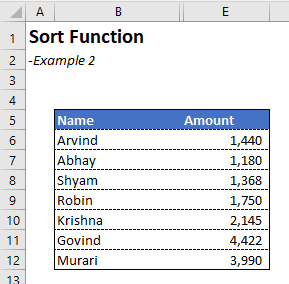To sort the list in descending order, we will use the first and third argument of the Sort function.

=Sort(B:6:B12,,-1). Press Enter.

We get the list sorted in descending order (see below).### Example 3 – Sorting a List of Names with sales made in descending order.

Here is the list of customers and sales made last week. We want to organize the data in descending order by total sales.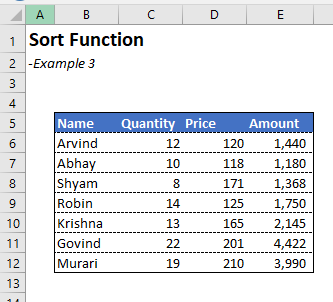To sort the data in descending order by total revenues, we will use the first 3 arguments of the sort function. Type

=Sort(B6:E12,4,-1)

B6:E12 is the range to be sorted.

4 is the column in the range to be sorted.

-1 is for descending order.

When we Press Enter. We get the following table sorted in descending order.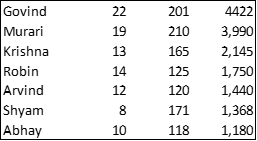If we type B5:B12 as the range. Excel recognizes that the first row is table header. It gives the following table.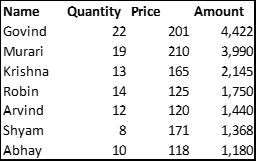### Example 4 – Sorting a List of Unique Names of Customers.

Here is a list of names of customers who visited the store during this month. Names of a few customers is appearing more than once in the list.

We are required to create a list of unique names sorted in alphabetical order.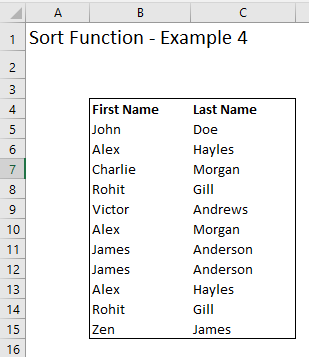To create a list of unique names sorted in alphabetical order, we will use the Unique and sort functions combined.

To create the unique list we will type.

=Unique(B5:C15)

We get this list of unique names.Then we will use the Sort function along with the Unique function to sort the list in alphabetical order.

Type

=Sort(Unique(B5:C15))

Press Enter.

Now we get the list sorted in alphabetical order.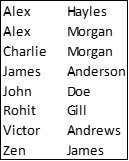To combine the first and last names, we can modify the formula a bit.

Type

=Sort(Unique(B5:B15&” “&C5:C15))

Press Enter

Now you get the sorted list with first and last names in the same column.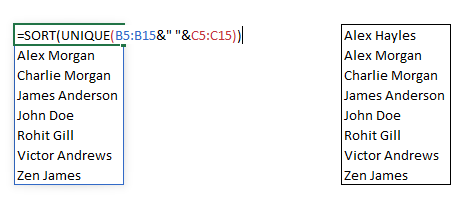This is how we can use the Sort function. It can be used for analyzing large data sets.

Click to learn data analysis with Pivot Tables

Let us know in case you have any questions, by posting in the comment section below.

Happy learning.

1.